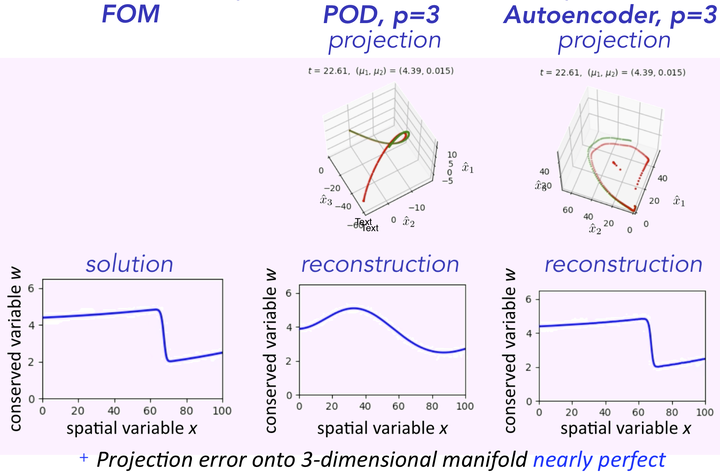# Model reduction of dynamical systems on nonlinear manifolds using deep convolutional autoencoders### Abstract

Nearly all model-reduction techniques project the governing equations onto a linear subspace of the original state space. Such subspaces are typically computed using methods such as balanced truncation, rational interpolation, the reduced-basis method, and (balanced) proper orthogonal decomposition (POD). Unfortu- nately, restricting the state to evolve in a linear subspace imposes a fundamental limitation to the accuracy of the resulting reduced-order model (ROM). In particular, linear-subspace ROMs can be expected to produce low-dimensional models with high accuracy only if the problem admits a fast decaying Kolmogorov n-width (e.g., diffusion-dominated problems). Unfortunately, many problems of interest exhibit a slowly decaying Kolmogorov n-width (e.g., advection-dominated problems). To address this, we propose a novel framework for projecting dynamical systems onto nonlinear manifolds using minimum-residual formulations at the time-continuous and time-discrete levels; the former leads to manifold Galerkin projection, while the latter leads to manifold least-squares Petrov–Galerkin (LSPG) projection. We perform analyses that provide insight into the relationship between these proposed approaches and classical linear-subspace reduced-order models. We also propose a computationally practical approach for computing the nonlinear manifold, which is based on convolutional autoencoders from deep learning. Finally, we demonstrate the ability of the method to significantly outperform even the optimal linear-subspace ROM on benchmark advection-dominated problems, thereby demonstrating the method’s ability to overcome the intrinsic n-width limitations of linear subspaces.

Date
Jul 29, 2019 —
Location
Austin, Texas